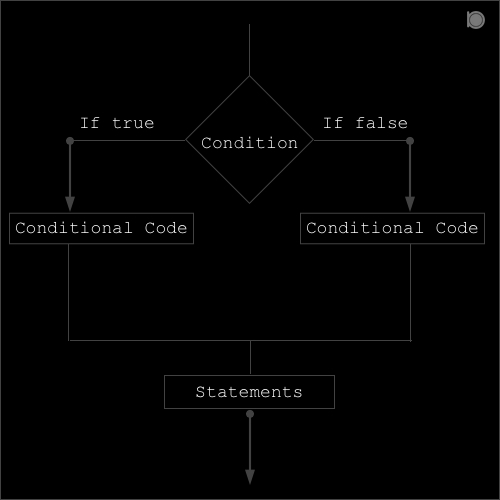If … else statement in C language contains two blocks true and false block which means if the specified condition is true then the true block is executed and if the condition is false then else (false) block is executed.##### Syntax of if … else statement:
``````if (condition or expression)
{
conditional code;
}
else
{
conditional code;
}
``````

### Program example of if … else statement in C

``````//An example of if ... else statement in C
#include<stdio.h>

int main()
{
int a = 10, b = 20;
printf("Consider a = 10 and b = 20\n");
if(a>b)
{
printf("a is greater\n");
}
else
{
printf("b is greater\n");
}

printf("This is an example of if ... else statement in C\n");
return 0;
}
``````

### Output

``````Consider a = 10 and b = 20
b is greater
This is an example of if statement in C
``````

In the above example we have two integer variables `a = 10 and b = 20`. Here in the if block we have a condition `a>b` since `a is smaller than b` so the condition is `false` so the `true block` is skipped and the `false (else) block` is executed.